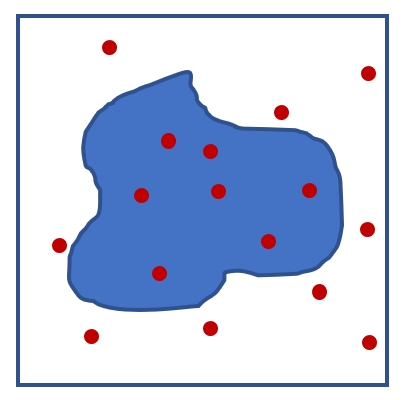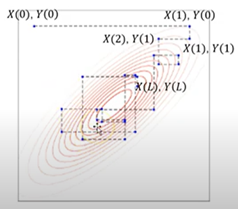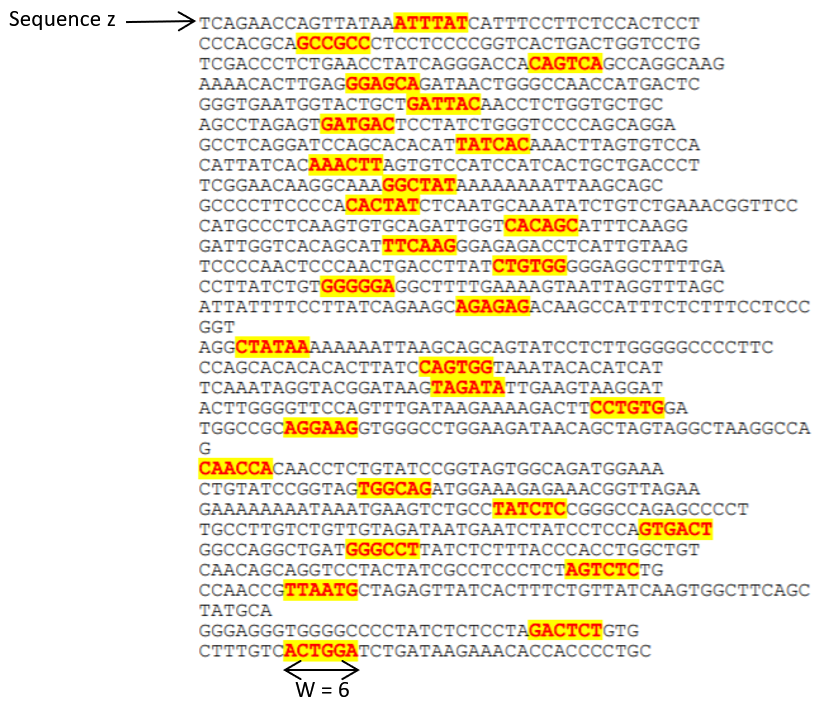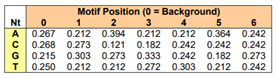# Markov chain Monte Carlo — Gibbs Sampling for DNA sequence alignment

The Markov chain Monte Carlo (MCMC) is a sampling method that allows us to estimate parameters of an intractable or unknown, possibly high dimensional (depends on many parameters) distribution by randomly sampling from a simpler distribution known as the proposal distribution. This is particularly useful when applying Bayesian statistics to obtain samples from an unknown or intractable posterior distribution (the revised probability distribution after accounting for the data). It is also useful for many biological applications involving large amounts of data that would be difficult to analyse otherwise.

In this article, we will give a short introduction to MCMC and one of the most general algorithms for MCMC known as Gibbs sampling. In particular, we will show how it can be applied to the problem of DNA sequence alignment — one of the fundamental problems in Biology. By identifying regions of the genome (or protein) that is repeated in many sequences, we can identify the structures that has been conserved and hence may be functionally important or may serve as potential targets for drug therapies. It is hoped that this article will help the reader to gain a more intuitive understanding for MCMC and why it is such a powerful and convenient numerical method.

The name MCMC is a combination of Monte Carlo and Markov chain. Monte Carlo refers to a class of methods that relies on random sampling to estimate numerical results. For example, we might want to find the area of some arbitrary shape. We first draw a box around the shape. This box can be thought of as the domain or the range of values that our points can take. We then randomly generate points in the box such that every point is equally likely.The Monte Carlo method allows us to estimate the size of the shaded area by counting the number of randomly generated points inside the area.

We can then approximate the ratio of the shaded area to the box as the number of points inside the shaded area over the total number of random points generated. As the number of random points generated increases, this approximation improves. This method works for arbitrarily complex shapes for which we are unable to solve for the area exactly.

Markov chains are stochastic models describing a sequence of possible states where the probability of the next state depends only on the current state. For example, there is a 0.4 probability of it raining tomorrow if it rains today (and hence 0.6 chance of it not raining tomorrow if it rains today), and a 0.2 chance of it raining tomorrow if it is not raining today (and hence 0.8 chance of it not raining tomorrow if it doesn’t rain today). There are, thus, 2 states in this model — raining (state 1) and not raining (state 2). It can be illustrated as follows:

The chain refers to the sequence of events linked by the arrows. We can write the probability of changing or transitioning from one state to another in the form of a matrix known as the transition matrix:

We can see that an important property of Markov chains is that the probability of transitioning to the next state depends only on the current state, i.e. whether it rains tomorrow depends only on whether it is raining today. This property is known as memorylessness. In the context of MCMC, this means that each random sample is generated based on the last random sample before it, and does not depend on any sample before that. 

A good analogy for MCMC is that of ants looking for food. Many ants leave the nest looking for crumbs and they leave behind a scent trail. Some of these ants will by chance head in the right direction to locate the food, and they will return to the nest, marking their scent trails to indicate the location of potential food. This scent trail indicates a rough direction and it might not be the optimal path to the food. Here we can think of the optimal path to food as the parameter to be estimated in the MCMC and the ants leaving the nest as random samples. When they hit close to the target by chance, they add ‘weight’ to that direction by leaving scent trails. That makes it more likely that other ants will follow that trail and further reinforces that trail. The ants do not follow the path strictly and eventually a more direct path might be found then the possibly meandering initial path to food. This is similar to the MCMC process where we try to explore the entire universe of possible solutions for the parameters to be estimated, and when we hit close to the solution, we then try to ensure that our future samples are refined to target the area close to the solution and eventually arrive at a good estimate of the solution.

One of the most common MCMC algorithm is ‘Gibbs Sampling’. Gibbs sampling can be used when given the conditional probabilities of the parameters of interest, we are interested in finding their joint unconditional probabilities. Conditional probabilities are probabilities that assume the satisfaction of certain conditions. In the raining day example, the conditional probability of it raining tomorrow, given that it is raining today, is 0.4; on the other hand, if it does not rain today, the conditional probability is 0.2. The unconditional probability of whether it rains would be the probability of it raining on any given day, which, in this simple example, would be:

P(raining tomorrow | raining today) x P(raining today) + P(raining tomorrow | not raining today) x P(not raining today)

Joint unconditional probability refers to the probability of multiple events happening together, for example: the probability on any day (regardless of yesterday’s weather) that it is raining and you brought an umbrella. This can be written as P(raining today, brought an umbrella).

We can imagine a scenario where we have 2 variables X and Y. We know that P(X|Y) is a normal distribution with some mean and standard deviation, and the probability of P(Y|X) is also a normal distribution with some mean and standard deviation. We now want to draw samples from their joint probability P(X,Y). Applying the Gibbs sampling algorithm, we can sample from P(X,Y) without ever explicitly calculating P(X,Y). We first start by assuming some value for X and Y. This is step 0 and the first ‘entry’ in the chain of samples. We will denote this as X(0) and Y(0). We now sample from P(X|Y=Y(0)) to get X(1). Using X(1) we sample the next value Y(1) using P(Y|X=X(1)). We repeat the sampling steps alternating between sampling X and Y. If we allow this sampling to run for a long enough time, the resulting samples will have the same distribution as the joint distribution P(X,Y), all without ever computing P(X,Y):Plot of samples drawn from 2 conditional normal distribution using Gibbs sampling. Source: Taken from 

We can extend this to models with many dependent variables for which obtaining the analytical expression for the distribution of P(x1,x2,…,xn) might be impractical.

We will now walk through the Gibbs sampler algorithm applied to a DNA sequence alignment to identify a single motif (a shorter sequence such as ‘ATTTAT’ within the whole DNA sequence that is recurring in many sequences, like a repeated pattern in a mosaic, a piece of music or your table cloth). First, we will count the numbers of each nucleotide A, T, C and G in all the sequences to be aligned. This allows us to determine the frequency of their occurrence in the sequences, and it is called the background frequency. We then randomly select a position for the motif within each sequence (highlighted in below Figure 4), and count the frequency of the nucleotides in each position in the randomly selected motifs; this will be referred to as motif frequency.Figure 4. Initial randomly initialised motif location. Source: Taken from 

One of the sequences (we will call it sequence z) is selected either randomly or sequentially. We select a new motif position for this sequence by sampling according to a weighted distribution. We first consider all the possible segments of predefined width W (width of the motif to be found). We calculate the probability of each possible segment of width W arising from random chance by taking the product of the background frequencies of each nucleotide in the sequence. For example,Figure 5. Nucleotide frequencies at different positions in motif. Source: Taken from 

In Figure 5 the background frequencies are A: 0.267, C: 0.268, G: 0.215 and T: 0.250. Then for a sequence ATTTAT, the probability of this sequence will be (0.267)(0.25)³(0.267)(0.250)=2.75E-4. We also calculate the probability of each sequence arising using the motif frequency which in Figure 5 would be (0.212)(0.212)(0.272)(0.303)(0.364)(0.242)=3.26E-4. We then calculate a weight for each sequence by taking the probability of each sequence calculated using the motif frequency divided by the probability of each sequence calculated using background probability, 3.26/2.78=1.17. A sample is then drawn according to this weighted distribution. Motifs with higher weights would thus have a higher chance of being sampled. After each sampling step we can update the motif frequency with the newly sampled motif. We then repeat this for all the sequences to be aligned. When we have sampled all the sequences, we can calculate the maximum posteriori (probability post observing the data) estimate by taking the sum of all the log (motif frequency/ background frequency) across all sampled motifs,

For nucleotide A at position 1 in the motif, the log-odds ratio is . We want to sum this across the whole motif and across all sequences. Example for ATTTAT it is . We have to sum this across all sequences, hence for the next sequences ‘GCCGCC’, ‘CAGTCA’ etc (see Figure 4).

Our goal is to maximise the maximum posteriori estimate. By iterating through this sampling and updating of motif frequency, it is hoped that when, by chance, some correct motif positions are selected, the different weighting will begin to favour the correct motifs and result in a more accurate alignment (Figure 7):

For a more detailed discussion of the method and sample results, the reader is advised to refer to the technical report by Eric Rouchka . This stochastic approach has approximately a linear time complexity, which means that the length of time it takes to run it increases roughly proportionally to the number of sequences to be aligned. This is in contrast to many deterministic expectation maximisation algorithms for aligning genetic sequences whose time complexities grow exponentially. It is also faster than algorithms that work by comparing sequence pairwise which have time complexities of at least quadratic.  This basic algorithm allows us to detect motif without any prior information. The basic algorithm has been improved to be able to incorporate additional prior knowledge such as phylogeny  and motif structure  to further optimise the performance for specific biological applications.

Aside from Gibbs sampling, many other algorithms exist for MCMC including Metropolis-Hastings and Hamilton . It has been applied to many different domains from drug discovery [10–12], epidemiology  to ecology . In conclusion, MCMC is a powerful way that allows us to estimate parameters from complex data. Typical of stochastic numerical methods, it does not guarantee the right or best answer, and there is no guarantee that the solutions converge. However, it gives us another tool to approximate solutions to otherwise intractable problems in a reasonable time. This paper outlined the general idea of how this can be accomplished, and illustrated how this has been applied to the important problem of DNA sequence alignment.

References

 D. van Ravenzwaaij, P. Cassey, and S. D. Brown, “A simple introduction to Markov Chain Monte–Carlo sampling,” Psychonomic Bulletin & Review, vol. 25, pp. 143–154, 2018/02/01 2018.

 M. Pyrcz, “11e Machine Learning: Markov Chain Monte Carlo,” ed. YouTube: https://www.youtube.com/watch?v=7QX-yVboLhk, 2019.

 X. Xia, “Bioinformatics and Drug Discovery,” Current Topics in Medicinal Chemistry, vol. 17, pp. 1709–1726, // 2017.

 E. C. Rouchka, “A brief overview of gibbs sampling,” Bioinformatics Technical Report Series, No. TR-ULBL-2008–02, University of Louisville, vol. 9, 1997.

 C. E. Lawrence, S. F. Altschul, M. S. Boguski, J. S. Liu, A. F. Neuwald, and J. C. Wootton, “Detecting subtle sequence signals: a Gibbs sampling strategy for multiple alignment,” Science, vol. 262, p. 208, 1993.

 R. Siddharthan, E. D. Siggia, and E. van Nimwegen, “PhyloGibbs: A Gibbs Sampling Motif Finder That Incorporates Phylogeny,” PLOS Computational Biology, vol. 1, p. e67, 2005.

 A. V. Favorov, M. S. Gelfand, A. V. Gerasimova, D. A. Ravcheev, A. A. Mironov, and V. J. Makeev, “A Gibbs sampler for identification of symmetrically structured, spaced DNA motifs with improved estimation of the signal length,” Bioinformatics, vol. 21, pp. 2240–2245, 2005.

 S. Chib and E. Greenberg, “Understanding the Metropolis-Hastings Algorithm,” The American Statistician, vol. 49, pp. 327–335, 1995/11/01 1995.

 R. M. Neal, “MCMC using Hamiltonian dynamics,” Handbook of markov chain monte carlo, vol. 2, p. 2, 2011.

 B. Jayawardhana, D. B. Kell, and M. Rattray, “Bayesian inference of the sites of perturbations in metabolic pathways via Markov chain Monte Carlo,” Bioinformatics, vol. 24, pp. 1191–1197, 2008.

 R. Frank and R. Hargreaves, “Clinical biomarkers in drug discovery and development,” Nature Reviews Drug Discovery, vol. 2, pp. 566–580, 2003/07/01 2003.

 M. Trägårdh, M. J. Chappell, A. Ahnmark, D. Lindén, N. D. Evans, and P. Gennemark, “Input estimation for drug discovery using optimal control and Markov chain Monte Carlo approaches,” Journal of pharmacokinetics and pharmacodynamics, vol. 43, pp. 207–221, 2016.

 G. Hamra, R. MacLehose, and D. Richardson, “Markov Chain Monte Carlo: an introduction for epidemiologists,” International Journal of Epidemiology, vol. 42, pp. 627–634, 2013.

 G. W. Cobb and Y.-P. Chen, “An Application of Markov Chain Monte Carlo to Community Ecology,” The American Mathematical Monthly, vol. 110, pp. 265–288, 2003/04/01 2003.

--

--